Courses

# Test: Phase Controlled Rectifiers- 2

## 15 Questions MCQ Test Topicwise Question Bank for Electrical Engineering | Test: Phase Controlled Rectifiers- 2

Description
This mock test of Test: Phase Controlled Rectifiers- 2 for Electrical Engineering (EE) helps you for every Electrical Engineering (EE) entrance exam. This contains 15 Multiple Choice Questions for Electrical Engineering (EE) Test: Phase Controlled Rectifiers- 2 (mcq) to study with solutions a complete question bank. The solved questions answers in this Test: Phase Controlled Rectifiers- 2 quiz give you a good mix of easy questions and tough questions. Electrical Engineering (EE) students definitely take this Test: Phase Controlled Rectifiers- 2 exercise for a better result in the exam. You can find other Test: Phase Controlled Rectifiers- 2 extra questions, long questions & short questions for Electrical Engineering (EE) on EduRev as well by searching above.
QUESTION: 1

### Consider the following statements associated with single phase full converters: 1. Mid-point converter configuration is used in case the terminals on dc side have to be grounded. 2. The transformer rating in mid-point converter is double the load rating. 3. SCRs are subjected to a peak inverse voltage of 2 Vm in single phase mid-point converter. 4. Bridge converter is preferred over mid-point converter. Which of these statements are correct?

Solution:

In mid-point converter, each secondary should be able to supply the load power. Due to this reason, the transformer rating in mid-point converter is double the load rating. Thus, statement-2 is not correct.

QUESTION: 2

### Assertion (A): The commutation overlap is more predominant is semiconverters than in full converters. Reason (R): The commutation period in seconds, when outgoing and incoming SCRs are conducting, is known as the overlap period.

Solution:

The commutation overlap is more predominant in full converter than in semi converter. Hence, assertion is a false statement.

QUESTION: 3

### For a single-phase full converter having a full load current of I0 match List - I (Overlap angle) with List-ll (Output voltage) and select the correct answer using the codes given below the lists (Ls = source inductance):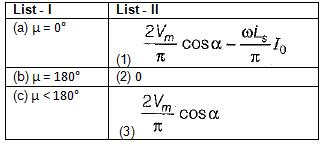Codes: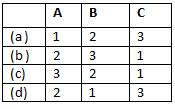Solution:

• In a single-phase full converter, as long as μ < 180°, the output voltage will be given by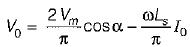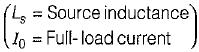• When μ = 180°, the load will be permanently short circuited by SCRs and the output voltage will be zero because during the overlap angle, all SCRs will be conducting.
•  Since,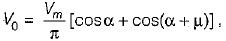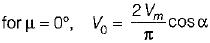QUESTION: 4

Which of the following converters can feed power in any of the four quadrants?

Solution:

A dual converter using two full converters can give four quadrant operation as shown below.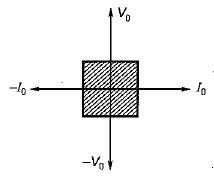QUESTION: 5

Consider the following statements:
1. In a full converter, direction of current cannot reverse.
2. Semi-converters are single quadrant converters.
3. A full converter can operate as a two-quadrant converter.
4. A full converter operates as a rectifier in first quadrant and as an inverter in the second quadrant.
5. In a full converter, direction of current cannot reverse but polarity of output voltage can be reversed.

Q. Which of the statements given above are correct?

Solution:

A full converter operates as a rectifier in first quadrant (both V0, I0 positive) from α = 0° to 90° and as an inverter (V0 positive but I0 negative) from α = 90° to 180° in the fourth quadrant as shown below.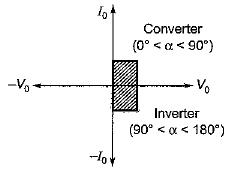Thus, statement-4 is not correct. Statements 1, 2,3 and 5 are correct.

QUESTION: 6

AC-to-DC circulating current dual converters are operated with which of the following relationship between their triggering angles (α1 and α2)?

Solution:

In AC-to-DC circulating current dual converters, the firing pulses of the two converters are so adjusted that α1 + α2 = 180°.

QUESTION: 7

A single-phase half-wave controlled converter with resistive load is operated from a 120 V, 50 Hz supply. If the average output voltage is 25% of the maximum possible output voltage, the firing angle of the thyristor would be equal to

Solution:

The average output voltage is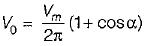The maximum output voltage is obtained when α = 0°
∴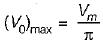According to question,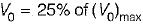or,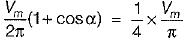or,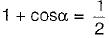or,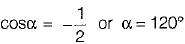QUESTION: 8

A single phase fully controlled thyristor bridge converter supplies a load consisting of R, L and E has shown in figure below. The inductance L in the circuit is so large that the output current may be considered to be virtually constant. The firing angle α is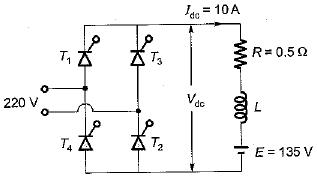Solution:

For a single-phase fully controlled thyristor bridge converter with R, L, E load, the average output voltage is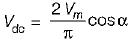or,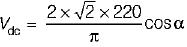= 198 cos α ....(i)
Now, Vdc - Idc R+ E- (10 x 0.5) + 135
or, Vdc = 140V    ...(ii)
From equations (i) and (ii),we have:
198 cos α = 140
or,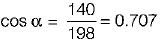or, Firing angle α = 45°

QUESTION: 9

Consider the following statements:
1. Fully-controlled and half controlled circuits both are capable of regeneration.
2. The higher the pulse number, the lower is the magnitude of ripple voltage and current in the load-circuit and the a.c. supply.
3. Bridge circuits and half-wave circuits both require same number of thyristors to carry the same current.
4. Half-wave circuits tend to be used for low-voltage, high-current use.Which of the statements given above is/are not correct?

Solution:

• Only fully controlled circuits are capable of inversion and therefore regeneration, while half-controlled circuits are not. Thus, statement-1 is not correct.
• Statement-2 is correct.
• Bridge circuits require twice as many thyristors than half-wave circuits to carry the same current. Thus, statement-3 is not correct.
• Statement-4 is correct.

QUESTION: 10

In a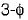bridge converter the dc load current is 90 A, the commutating inductance per phase is 50 and thepower supply is 2.3 kV, 50 Hz. The reduction in output voltage due to overlap is around

Solution:

The reduction in output voltage due to overlap is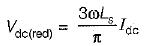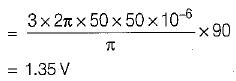QUESTION: 11

The holding current of SCRs in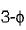full-converter is 200 mA and the delay time is 2.5 p.sec. Converter is supplied from a star-connected 208 V, 50 Hz supply, has a load of R = 2 Ω L = 8 mH and the firing angle is 60°. The minimum width of gate pulse tp for L = 0 H is

Solution:

Given,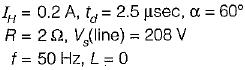∴ Phase voltage,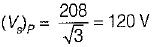and peak voltage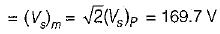Now,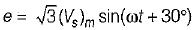At ωt = 60°, e = √3x169.7sin90° ≈ 293.93V
Rate of rise of anode current, di/dt at the instant of triggering is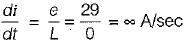∴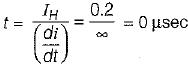∴ Minimum width of gate-pulse is
tp = t + td = 0 + 2.5 = 2.5μs

QUESTION: 12

The nature of load current, l.e., whether load is continuous or discontinuous in controlled rectifiers

Solution:

The term continuous means that load current never ceases but continues to flow through SCR or their combination. The term discontinuous is applied to the condition when load current reaches zero during each half cycle before the next SCR in sequence is fired. In practice, the output current may become discontinuous at high values of firing angle or at low values of load current.

QUESTION: 13

In a 3-phase full converter, the output voltage during overlap is equal to

Solution:

Output voltage with no overlap = internal voltage of the 3-phase full converter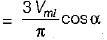Output voltage with overlap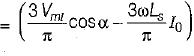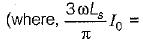Average value of fall in output voltage due to overlap)

QUESTION: 14

The peak inverse voltage in ac to dc converter system is highest in

Solution:

PIV of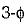bridge converter =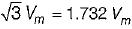PIV ofhalf wave converter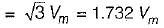PIV of single-phase full-wave mid-point converter
= - 2 Vm (Highest)
PIV of single-phase full-converter = - Vm

QUESTION: 15

A transformer is having source voltage of 400 volt and turns ratio of 2:1. The transformer is centre-tapped. If the secondary is connected to single phase full-wave converter, PIV per SCR will be

Solution:

Secondary voltage =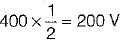∴ PIV = 200√2 V